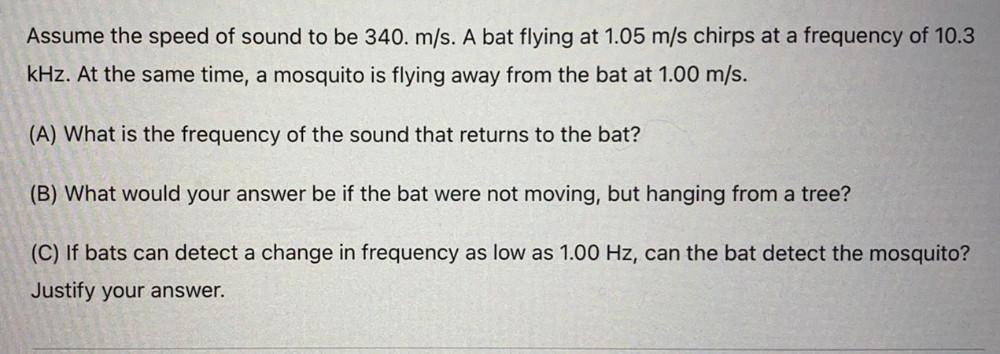Question:

# Assume the speed of sound to be 340. m/s. A bat flying at 1.05 m/s chirps at a frequency of 10.3 kHz. At the same time, a mosquiAssume the speed of sound to be 340. m/s. A bat flying at 1.05 m/s chirps at a frequency of 10.3 kHz. At the same time, a mosquito is flying away from the bat at 1.00 m/s. (A) What is the frequency of the sound that returns to the bat? (B) What would your answer be if the bat were not moving, but hanging from a tree? (C) If bats can detect a change in frequency as low as 1.00 Hz, can the bat detect the mosquito? Justify your answer.